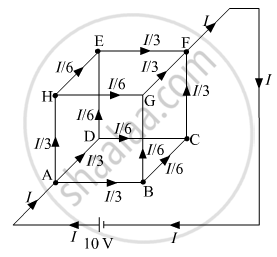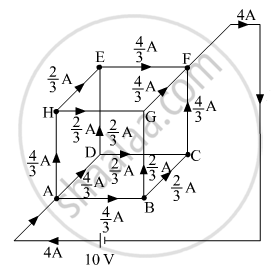# Twelve wires each having a resistance of 3 Ω are connected to form a cubical network. A battery of 10 V and negligible internal resistance is connected across the diagonally opposite - Physics

Twelve wires each having a resistance of 3 Ω are connected to form a cubical network. A battery of 10 V and negligible internal resistance is connected across the diagonally opposite corners of this network. Determine its equivalent resistance and the current along each edge of the cube.

#### Solution

A battery of 10 V is connected across the diagonally opposite points a and f of the given network. Each side of the cube represents a 3-ohm resistor. Current entrees through the point a and leaves through the point f. Let I current is drawn from the battery and enters through point a, due to the symmetry of cube between point a and f, the current will divide itself into equal parts at each corner of the cube.
As you can see in the figure given below:If we consider the current path ABGFA, then we can write, VA-B + VB-G  + VG-F = V

⇒ ("I"/3 xx"R") + ("I"/6 xx "R") + ("I"/3 xx "R") = ("I" xx "R"_"net")

⇒ "R"_"net" = "R"/3 + "R"/6 + "R"/3 = (5"R")/6

∵ R = 3Ω

⇒ "R"_"net" = (5xx3)/6 = 2.5 Ω

Current, "i" = "V"/"R"_"net" = 10/2.5 = 4 "A"

⇒ i = 4 A

As i = 4 A, hence current through each side of the cube can be marked. The below figure shows the current through each resistance of the network. i.e. each side of the cube.Concept: Kirchhoff’s Rules
Is there an error in this question or solution?